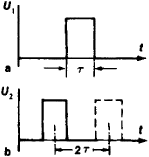# Coincidence Method

## Coincidence Method

in metrology, a measurement technique involving comparison with a standard. In the coincidence method, the difference between the quantity being measured and the quantity reproduced by the standard is measured according to the coincidence of marks on a scale or of periodic signals. An example is the measurement of length by means of a vernier caliper; such measurement is based on the coincidence of the divisions on the main scale and the vernier. Another example is the determination of the period of pulsars; this measurement is based on the coincidence of the pulse maxima of the pulsar radiation with the marks of equal time intervals on a chronograph chart.

## Coincidence Method

(or coincidence counting), a method of investigation widely used in nuclear physics. It is based on the use of coincidence circuits and permits establishment of the time relations between various correlating events. Thus, in the study of elementary nuclear-interaction events (for example, interactions between atomic nuclei and high-energy particles produced in charged-particle accelerators) one of the principal methods of establishing the sequence of appearance of secondary particles and gamma quanta is to register coincident electrical signals from nuclear radiation detectors. Here, signals that fully or partially overlap in time (Figure 1) are said to be coincident.Figure 1. Coincidence pulses: (a) input pulse in first channel, (b) limiting positions of input pulse in second channel for pulses in the two channels to be regarded as coincident; (U) pulse amplitude, (τ) pulse duration, (t) time

The practical use of the coincidence method presupposes knowledge of the coincidence curve—that is, the dependence of the number of coincidence-circuit output signals on the time interval Δt between input electrical signals. For a two-channel (two-input) coincidence circuit with rectangular input signals of duration τ, the coincidence curve, in the ideal case, also has a rectangular shape. Because of noise and the influence of various statistical factors, under real conditions the coincidence curve may assume the shape of a normal-distribution curve. The width of the curve at half its maximum height is called the resolving time Tres and corresponds to the maximum value of Δt between two events that satisfy the simultaneity condition. The resolving time is determined by the triggering threshold of the coincidence circuit.

By means of the coincidence method, the effect of false coincidences on the registration of nuclear interactions can be sharply reduced. Such coincidences arise because there usually is a large flux of background signals in addition to the events under study. As an example, let us consider the case where the background signals occur randomly. For one detector, the average number of events (signals) per unit time is v1, and the duration of the signals is τ1. For the other detector, the corresponding quantities are v2and τ2. The number of randomly coincident signals from the two detectors is then equal to nrandomv1v21 + τ2). This number is proportional to the mutual overlap time of the signals. Use of the m-fold coincidence method gives the number of random coincidences nrandommv1 ... vm τm –1 (when vτ < 1 and τ1 = τ2 = ... = τm). The time intervals τ are usually between 10–9 and 10–5 sec long.

### REFERENCES

Gol’danskii, V. I., A. V. Kutsenko, and M. I. Podgoretskii. Statistika otschetovpri registratsii iadernykh chastits. Moscow, 1959.
Kowalski, E. Iadernaia elektronika. Moscow, 1972. (Translated from English.)
Rekhin, E. I., and P. S. Chernov. Metodsovpadenii. Moscow, 1976.

I. V. SHTRANIKH

Site: Follow: Share:
Open / Close